Worksheets and No Prep Teaching Resources
Math Worksheets

# Subtraction Worksheets

Students will "take away" great skills in subtraction with these exciting worksheets! Greater mastery in subtraction, a basic math skill, comes through varied worksheets from two digits up to six digits for whole numbers, fractions, and decimals. Problems use both column and across formats. Help your mathematicians become proficient in problems with regrouping and other skills in the enriching subtraction math centers!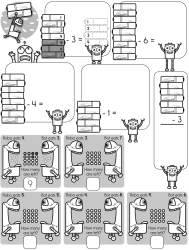Subtraction Scoot Cards

Subtraction Math Facts

Quick Math Facts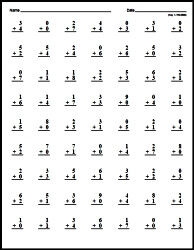Make Quick Math Facts Printable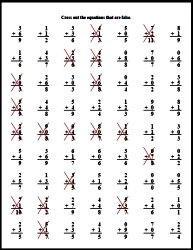Subtraction Math Center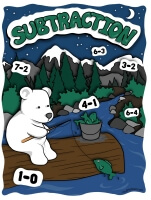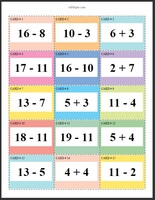Subtraction Math Center: Differences of 2 to 9 (Grades 3-6) Subtraction Math Center: Differences of 2 to 19 (Grades 3-6)

Addition and Subtraction Math Center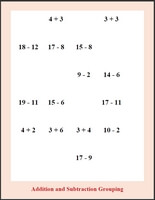Sums and Differences of 2 to 9 (Grades 3-6) Sums and Differences of 2 to 19 (Grades 3-6)

Subtraction Stairs Math Learning Center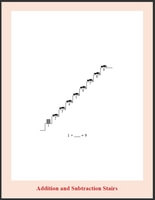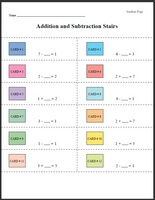Subtraction Stairs: Numbers 1 to 5 (Grades 1-3) Subtraction Stairs: Numbers 1 to 9 (Grades 1-3) Subtraction Stairs: Numbers 1 to 14 (Grades 1-3) Subtraction Stairs: Numbers 1 to 19 (Grades 1-3)

Color by Subtraction

Introduction to Subtraction

Subtracting a Fixed Number - Number line is from 0 to 9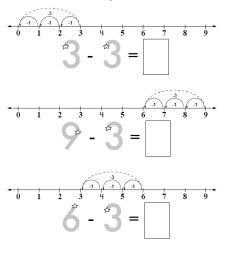Subtracting 1 Subtracting 2 Subtracting 3 Subtracting 4 Subtracting 5 Mixed - No Focus Number

Subtracting a Fixed Number - Number line is from 0 to 9 - Last Problem No Arrows (just the numberline)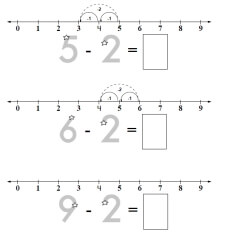Subtracting 1 Subtracting 2 Subtracting 3 Subtracting 4 Subtracting 5 Mixed - No Focus Number

Subtracting a Fixed Number - Number line is from 0 to 19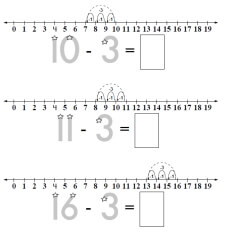Subtracting 1 Subtracting 2 Subtracting 3 Subtracting 4 Subtracting 5 Subtracting 6 Subtracting 7 Subtracting 8 Mixed - No Focus Number

Subtracting a Fixed Number - Number line is from 0 to 9 - One Number Line (for first problem only)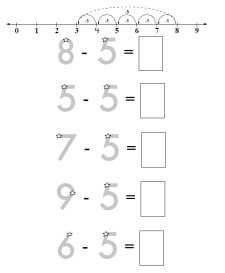Subtracting 1 Subtracting 2 Subtracting 3 Subtracting 4 Subtracting 5

Subtraction Challenge - Blanks in Different Spots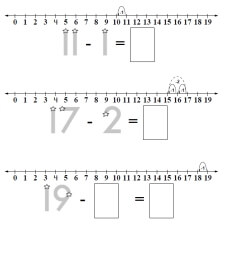Subtraction Challenge (number line from 0 to 9) Subtraction Challenge (number line from 0 to 19)

Subtraction

Subtraction Facts: Numbers 0 to 9
Subtraction Facts: Numbers 0 to 9
Large Fonts (fewer problems)
Small Fonts (more problems)

Subtraction Facts: Numbers 0 to 9 - Cross out the incorrect facts
Subtraction Facts: Numbers 0 to 9 - Cross out the incorrect facts
Large Fonts (fewer problems)
Small Fonts (more problems)

Subtraction Facts: Focusing on a number
Subtraction with the number 1
Subtraction with the number 2
Subtraction with the number 3
Subtraction with the number 4
Subtraction with the number 5
Subtraction with the number 6
Subtraction with the number 7
Subtraction with the number 8
Subtraction with the number 9

2-Digit Subtraction Facts
2-Digit subtraction
2-Digit subtraction with no regrouping
2-Digit subtraction with all regrouping

Runaway Math Puzzles
 Runaway Math Puzzles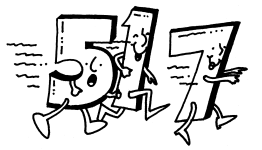Length: With Addition and Subtraction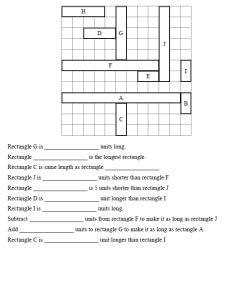Length: With Addition and Subtraction

Simple Subtraction Facts Activity Book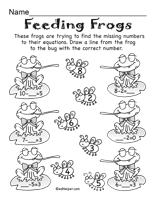Subtraction Puzzles

Easy Addition and Subtraction Activity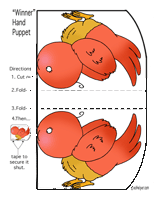A Winner of a Dinner

Subtraction
2 digits column subtraction
3 digits column subtraction
2-3 digits column subtraction
4 digits column subtraction
5-6 digits column subtraction
3-5 digits column subtraction
2 digits across subtraction
2-3 digits across subtraction
2-4 digits across subtraction
3-4 digits across subtraction
5-6 digits across subtraction
Column subtraction: start with 2 digits and increase by 1 digit in each row
Column subtraction: start with 2-3 digits and increase by 1 digits every 2 rows
Column subtraction: start with 2-3 digits and increase by 2 digits every 2 rows
Across subtraction with 3-5 numbers
Fill in the missing numbers: 2 digits
Fill in the missing numbers: 2-3 digits
Fill in the missing numbers: 4-5 digits
Fill in the missing numbers: 2-6 digits
Math word puzzles
Math addition boxes: 3 x 3
Math addition boxes: 4 x 4
Subtraction word problems (Easier)
Subtraction word problems
Subtraction Mixed Review

Bulletin Board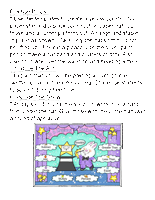"Be A Magical Mathematician" Bulletin Board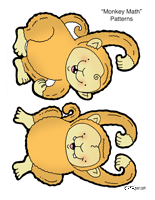Monkey Math

Math Equations Bulletin Board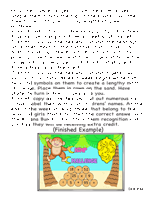"Chimp Challenge" Bulletin Board

Subtraction: Numbers 0 to 10
Subtracting the number 1
Subtracting the number 2
Subtracting the number 3
Subtracting the number 4
Subtracting the number 5
Subtracting the number 6
Subtracting 7-10
Subtracting 1-2
Subtracting 2-4
Subtracting 2-5
Subtracting 1-9

Fill in the missing numbers
Subtracting 1-3
Subtracting 2-4
Subtracting 2-5
Subtracting 4-7
Subtracting 3-8
Subtracting 1-9

Find subtraction facts
Word search: subtract the number 1
Word search: subtract the number 2
Word search: subtract the number 3
Word search: subtract the number 4
Word search: subtract the number 5
Word Search: subtracting 1-3
Word Search: subtracting 3-5
Word Search: subtracting 4-9

Word problems and review
Subtraction word problems
Subtraction (Numbers 0-10) Mixed Review

Subtraction Through 20
Subtraction with Blocks: subtracting 1-5 (includes the answer)
Find the difference
Fill in one number
Complete the subtraction fact

Subtraction with Blocks: subtracting 1-5
Find the difference
Fill in one number
Complete the subtraction fact

Subtraction with Blocks: subtracting 6-10 (includes the answer)
Find the difference
Fill in one number
Complete the subtraction fact

Subtraction with Blocks: subtracting 6-10
Find the difference
Fill in one number
Complete the subtraction fact

Comparing addition and subtraction
Subtracting 1 to 4 from 10 to 19
Subtracting 5 to 9 from 10 to 19
1 addition and 2 subtraction facts
1 subtraction and 2 addition facts
Mixed

Subtracting from 16 through 20
Horizontal and vertical subtraction
Vertical subtraction
Horizontal subtraction
Fill in the missing numbers
Word search
Word problems

Comparing addition and subtraction
Fill in the missing numbers (number is same for each set)
Fill in the missing numbers (number is same for each set)

Subtracting from 11 through 20
Horizontal and vertical subtraction
Vertical subtraction
Horizontal subtraction
Fill in the missing numbers
Word search
Word problems

Review
Subtraction Through 20 Mixed Review

Subtracting Two-Digit Numbers (No Regrouping)
Subtracting a one-digit number from a two-digit number (No Regrouping)
Subtracting tens and ones with blocks
Vertical subtraction - 2 digits on top and 1 digit on bottom
Fill in the missing number in each problem
Subtracting cents
Horizontal and vertical subtraction
Vertical subtraction
Horizontal subtraction
Fill in the missing numbers

Two-Digit Subtraction (No Regrouping)
Subtracting tens and ones with blocks
Vertical subtraction
Fill in the missing number in each problem
Subtracting cents
Horizontal and vertical subtraction
Vertical subtraction
Horizontal subtraction
Fill in the missing numbers

Word problems and review (No Regrouping)
Two-Digit subtraction word problems
Two-Digit Subtraction Mixed Review (No Regrouping)

Introduction to 2-Digit Subtraction with Regrouping
Regroup a number (with blocks)
Regroup a number
Do you need to regroup? (includes a number line)
Do you need to regroup?
Subtract one digit from two digits and then add to check (includes numbers in addition formula)
Subtract one digit from two digits and then add to check
Subtract one digit from two digits and then add to check (includes problems with no regrouping)
Subtract one digit from two digits and then add to check (does not include "What is")
2-digit subtraction
2-digit subtraction and then add to check
2-digit subtraction with cents
2-digit subtraction
Problem solving (with subtraction equation)
Introduction to 2-digit Addition with Regrouping Mixed Review

2-Digit Subtraction with Regrouping
Subtract 1-digit from 2-digits
Fill in the missing number: 1-digit from 2-digits
Subtracting cents: 1-digit from 2-digits
Subtracting cents: 2-digit subtraction
2-digit subtraction
Fill in the missing number: 2-digit subtraction
Problem solving
2-digit Subtraction with Regrouping Mixed Review

3-Digit Subtraction
2-Digits from 3-Digits
Subtract and then add to check (numbers included in addition fact)
Subtract and then add to check
Subtract
Subtract (column headers, but no regrouping boxes)
Fill in the missing numbers
Subtract money
3-Digits
Subtract and then add to check
3-digit subtraction (with column headers)
Subtract money
3-digit subtraction
Fill in the missing numbers
3-digit Subtraction Mixed Review

Add and Subtract Decimals
Column addition of 2 numbers (up to tenths)
Column addition of 2 numbers (up to hundredths)
Column addition of 2 numbers (up to thousandths)
Money column addition (up to \$99.99)
Money column addition (up to \$99,999.99)
Across addition of 2 numbers (up to hundredths)
Across addition of 2 numbers (up to thousandths)

Subtraction
Column subtraction of 2 numbers (up to tenths)
Column subtraction of 2 numbers (up to hundredths)
Column subtraction of 2 numbers (up to thousandths)
Money column subtraction (up to \$99.99)
Money column subtraction (up to \$99,999.99)
Across subtraction of 2 numbers (up to hundredths)
Across subtraction of 2 numbers (up to thousandths)

Mix of Addition and Subtraction
Column addition and subtraction of 2 numbers (up to tenths)
Column addition and subtraction of 2 numbers (up to hundredths)
Column addition and subtraction of 2 numbers (up to thousandths)
Money column addition and subtraction (up to \$99.99)
Money column addition and subtraction (up to \$99,999.99)
Across addition and subtraction of 2 numbers (up to hundredths)
Across addition and subtraction of 2 numbers (up to thousandths)

Part 2
Column addition of 3 numbers
Order of Operations (no parenthesis)
Order of Operations
Order of Operations (one missing number)
Order of Operations (one missing operation)
Order of Operations (fill in all numbers)
Order of Operations (fill in all operations)
Fill in the missing numbers (up to hundredths)
Fill in the missing numbers (up to thousandths)
Math box
Decimal addition and subtraction word problems
Addition and subtraction of decimals: Mixed Review

Subtraction from Number Word Searches!
Subtraction with numbers 1-6   - with number words    (Fill-In Puzzle)
Subtraction with numbers 1-9   - with number words    (Fill-In Puzzle)
Subtraction with the number one   - with number words    (Fill-In Puzzle)
Subtraction with the number two   - with number words    (Fill-In Puzzle)
Subtraction with the number three   - with number words    (Fill-In Puzzle)
Subtraction with the number four   - with number words    (Fill-In Puzzle)
Subtraction with the number five   - with number words    (Fill-In Puzzle)
Subtraction with the number six   - with number words    (Fill-In Puzzle)
Subtraction with the number seven   - with number words    (Fill-In Puzzle)
Subtraction with the number eight   - with number words    (Fill-In Puzzle)
Subtraction with the number nine   - with number words    (Fill-In Puzzle)
Subtraction with the number ten   - with number words    (Fill-In Puzzle)
Subtraction with the number eleven   - with number words    (Fill-In Puzzle)
Subtraction with numbers 1-13   - with number words    (Fill-In Puzzle)
Subtraction with numbers 1-13 (No Carrying)   - with number words    (Fill-In Puzzle)
Subtraction with numbers 10-20   - with number words    (Fill-In Puzzle)
Subtraction with numbers 10-20 (No Carrying)   - with number words    (Fill-In Puzzle)
Subtraction with numbers 1-20   - with number words    (Fill-In Puzzle)
Subtraction with numbers 1-20 (No Carrying)   - with number words    (Fill-In Puzzle)
2 Digit Subtraction   - with number words    (Fill-In Puzzle)
2 Digit Subtraction (No Carrying)   - with number words    (Fill-In Puzzle)
3 Digit Subtraction   - with number words
3 Digit Subtraction (No Carrying)   - with number words
2 and 3 Digit Subtraction   - with number words
2 and 3 Digit Subtraction (No Carrying)   - with number words
4 Digit Subtraction   - with number words
4 Digit Subtraction (No Carrying)   - with number words
3 and 4 Digit Subtraction   - with number words
3 and 4 Digit Subtraction (No Carrying)   - with number words
5 Digit Subtraction   - with number words
5 Digit Subtraction (No Carrying)   - with number words
1-5 Digit Subtraction   - with number words
1-5 Digit Subtraction   - with number words

Subtraction from Math Word Puzzles!
Subtraction Worksheets
Subtraction (no hints)
Subtraction (hints)
Subtraction (half the problems include a hint)

Subtraction Worksheets
The vowels are replaced with numbers!

Subtraction (no hints)
Subtraction (hints)
Subtraction (half the problems include a hint)

Subtraction Worksheets
Only the words are given. The order of the words are not given!

Subtraction (no hints)
Subtraction (hints)
Subtraction (half the problems include a hint)

Subtraction Worksheets
The vowels are replaced with numbers but the order of the words are not given!

Subtraction (no hints)
Subtraction (hints)
Subtraction (half the problems include a hint)

Subtraction from Missing Digits
Subtraction Missing Digits (1 Digit)
Subtraction Missing Digits (2 Digits)
Subtraction Missing Digits (1-2 Digits)
Subtraction Missing Digits (3 Digits)
Subtraction Missing Digits (2-3 Digits)
Subtraction Missing Digits (4 Digits)
Subtraction Missing Digits (3-4 Digits)
Subtraction Missing Digits (2-4 Digits)

Decimal Subtraction Missing Digits Worksheets
Decimal Subtraction Missing Digits (1 Digit, Decimal Places: 1 Digit)
Decimal Subtraction Missing Digits (1 Digit, Decimal Places: 2 Digits)
Decimal Subtraction Missing Digits (1 Digit, Decimal Places: 1-2 Digits)
Decimal Subtraction Missing Digits (1 Digit, Decimal Places: 3 Digits)
Decimal Subtraction Missing Digits (1 Digit, Decimal Places: 1-3 Digits)
Decimal Subtraction Missing Digits (2 Digits, Decimal Places: 1 Digit)
Decimal Subtraction Missing Digits (2 Digits, Decimal Places: 2 Digits)
Decimal Subtraction Missing Digits (2 Digits, Decimal Places: 1-2 Digits)
Decimal Subtraction Missing Digits (2 Digits, Decimal Places: 3 Digits)
Decimal Subtraction Missing Digits (2 Digits, Decimal Places: 1-3 Digits)
Decimal Subtraction Missing Digits (1-2 Digits, Decimal Places: 1 Digit)
Decimal Subtraction Missing Digits (1-2 Digits, Decimal Places: 2 Digits)
Decimal Subtraction Missing Digits (1-2 Digits, Decimal Places: 1-2 Digits)
Decimal Subtraction Missing Digits (1-2 Digits, Decimal Places: 3 Digits)
Decimal Subtraction Missing Digits (1-2 Digits, Decimal Places: 1-3 Digits)
Decimal Subtraction Missing Digits (3 Digits, Decimal Places: 1 Digit)
Decimal Subtraction Missing Digits (3 Digits, Decimal Places: 2 Digits)
Decimal Subtraction Missing Digits (3 Digits, Decimal Places: 1-2 Digits)
Decimal Subtraction Missing Digits (3 Digits, Decimal Places: 3 Digits)
Decimal Subtraction Missing Digits (3 Digits, Decimal Places: 1-3 Digits)
Decimal Subtraction Missing Digits (2-3 Digits, Decimal Places: 1 Digit)
Decimal Subtraction Missing Digits (2-3 Digits, Decimal Places: 2 Digits)
Decimal Subtraction Missing Digits (2-3 Digits, Decimal Places: 1-2 Digits)
Decimal Subtraction Missing Digits (2-3 Digits, Decimal Places: 3 Digits)
Decimal Subtraction Missing Digits (2-3 Digits, Decimal Places: 1-3 Digits)
Decimal Subtraction Missing Digits (1-3 Digits, Decimal Places: 1 Digit)
Decimal Subtraction Missing Digits (1-3 Digits, Decimal Places: 2 Digits)
Decimal Subtraction Missing Digits (1-3 Digits, Decimal Places: 1-2 Digits)
Decimal Subtraction Missing Digits (1-3 Digits, Decimal Places: 3 Digits)
Decimal Subtraction Missing Digits (1-3 Digits, Decimal Places: 1-3 Digits)

Subtraction from Number Puzzles!
* The following worksheets are for edHelper.com subscribers.
A subscription to edHelper includes access to the math, spelling, and vocabulary subscription areas. Sign up for the subscriber materials.
Numbers 0 to 6 (Top Down)
Numbers 0 to 6 (Across)
Numbers 7 to 19 (Top Down)
Numbers 7 to 19 (Across)
Numbers 0 to 13 (Top Down)
Numbers 0 to 13 (Across)
Numbers 0 to 20 (Top Down)
Numbers 0 to 20 (Across)
Numbers 0 to 29 (Across)
2 Digit Subtraction (No Borrowing)
2 Digit Subtraction (Borrowing)
3 Digit Subtraction (No Borrowing)
3 Digit Subtraction (Borrowing)
4 Digit Subtraction
Subtraction
Subtraction (Across and Top Down)
Subtraction (2 - 5 Digit Numbers)
Subtraction (Large Numbers)
Addition and Subtraction Word Problems (easy)
Addition and Subtraction Word Problems (easy)

Subtraction Sequences from Sequences Puzzles!
Addition and Subtraction Sequences
Addition and Subtraction of Decimals
Subtraction of Fractions

Subtraction of Fractions from Fraction Worksheets!
Subtraction of Fractions
Subtracting Fractions (Across)
Subtracting Fractions (Top Down)
Subtracting Fractions (Top Down: Harder)
Subtracting Fractions
Subtraction (Like Denominators)
Subtraction (Like Denominators- Reduce to Lowest Terms)
Subtraction (Different Denominators)
Subtracting Algebraic Fractions
Subtracting Algebraic Fractions (More Difficult)

Subtraction from Logic Puzzles!
* The following worksheets are for edHelper.com subscribers.
A subscription to edHelper includes access to the math, spelling, and vocabulary subscription areas. Sign up for the subscriber materials.
Pens (Small Numbers)
Stickers

Subtraction Word Problems from the Math Standardized Test Prep Section
* This section is for edHelper.com subscribers. A subscription to edHelper includes access to the math, spelling, and vocabulary subscription areas. Sign up for the subscriber materials.

 Subtraction Sheet #1 Sheet #2 Sheet #3 Sheet #4 Sheet #5 Sheet #6 Sheet #7 Sheet #8 Sheet #9 Review Sheet

Addition and Subtraction: Stickers

Build Worksheet - 4 People, 5 People, 6 People, 7 People, 8 People

Matthew, Kyle, Anna, and Andrew each started a sticker collection in January. Each one of them collected a different number of stickers in January and February. During the 1st month, they collected 27, 24, 31, and 22 stickers. During the 2nd month, they collected 43, 44, 47, and 45 stickers.

Figure out how many stickers each person collected in January and February.

 1 Matthew and Anna both were not the ones who collected twenty-seven stickers in January. 2 If Anna did not collect stickers in February then Anna would only have 47 stickers. 3 Andrew collected sixteen more stickers in February than in January. 4 Kyle has a total of sixty-nine stickers. 5 If Matthew did not collect stickers in January then Matthew would only have 31 stickers.

Pens

Build Worksheet - 4 People, 5 People, 6 People, 7 People, 8 People, 9 People

A Subtraction Sentence Making Activity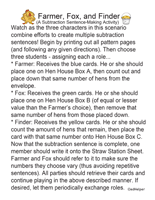Farmer, Fox, and Finder

More Activities and Printables
 Differences

Have a suggestion or would like to leave feedback?